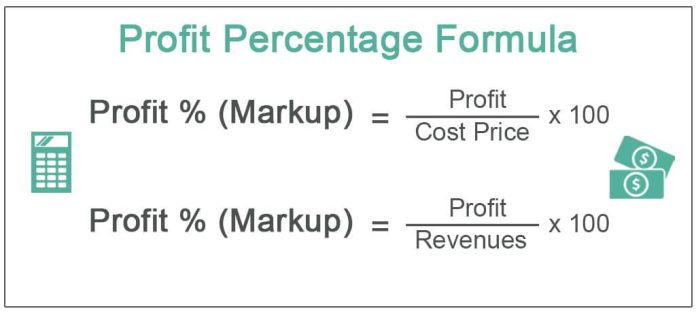# Understanding How Percentage Calculators Work and the Different Formulas

0Most people ignore the percentage symbol on electronic math calculators simply because they don’t know how to use it. Of course, it is a lengthy process. For instance, if you want to know what is 15% of 600 you have to key in 600 x 15 and then press the percentage symbol. You will get the results as 90, meaning that 15% of 600 is 90.

You might have found this procedure confusing, or you are uncertain of what you are doing. However, there is a convenient way of getting these values and their percentages; with a percentage calculator, you can conveniently key in any two values and get the results. This article examines how a percentage calculator works and the formulas used.

## What a Percentage Calculator Can Do

### You Can Directly Input Two Values to Get the Third Value

Unlike standard electronic calculators, you have to key in various expressions to get their percentages or what a particular percentage represents. A percentage calculator has a single equation with two inputs and the results. These buttons are used interchangeably depending on the value you are looking for.

### It Is Possible To Calculate Percentages from Common Phrases

Other than computing individual numeric values, a percentage calculator has predefined phrases where you can simply key in the values you want and click the “calculate button” to get the result; This further helps you the interpretation of mathematical expressions so that you don’t key in values in the wrong inputs.

### You Can Calculate Percentage Differences and Percentage Change

Sometimes, you would like to determine what a specific change in percentages depicts; it can be a drop or an increase. For instance, you would like to know the percentage decrease if a company’s total production capacity drops from 300 tons to 200 tons. All these operations are possible with a percentage calculator.

## Various Formulas Used In a Percentage Calculator

### The Percentage Formula

In whichever way you write a formula for percentage, it is usually a three-value algebraic expression V1 × p = V2 p represents the percentage and v1 and v2 and the first and the second values. The calculator will automatically convert p into a floating output as a percentage when displaying the results.

### The Formula For Calculating Percentage Difference

The calculator gets the percentage difference by finding the difference between the two values dividing the absolute value by the average sum of the two values and then multiplying the value obtained by 100.

### The Formula For Calculating Percentage Change

The percentage decrease or increase is usually calculated by getting the difference between the two values and comparing the change with the initial value. The calculator simply divides the absolute value (the difference between the two values) from the initial value and expresses it as a percentage.

## Summary

A percentage calculator enables you to find the percentages of values without necessarily writing any expression on paper. It is coded with formulas that allow you to find percentages of numbers, percentage differences, and percentage changes.